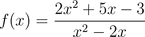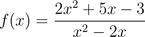# Horizontal Asymptote by Long Division?

• Painguy

## Homework Statement

Find the horizontal asymptote## The Attempt at a Solution

There are two ways I did this problem. One way seems to be a coincidence and the other the "proper way"

1. I used long division, and got 2+(9x-3)/(x^2-2x)
2 seems to be the horizontal asymptote of the function

2.If we remove the highest degree terms from the function you end up with (2+(5/x) -(3/x))/(-2/x) with 2 being the horizontal asymptote.

Which is the proper method and why is it that everything I've ran into so far displays this.

Painguy said:

## Homework Statement

Find the horizontal asymptote## The Attempt at a Solution

There are two ways I did this problem. One way seems to be a coincidence and the other the "proper way"

1. I used long division, and got 2+(9x-3)/(x^2-2x)
2 seems to be the horizontal asymptote of the function

2.If we remove the highest degree terms from the function you end up with (2+(5/x) -(3/x))/(-2/x) with 2 being the horizontal asymptote.

Which is the proper method and why is it that everything I've ran into so far displays this.

Both are correct methods, but you would probably choose to do the second method because it's easier (unless the question specifically asked for you to solve it with method 1.).
Do you understand why method 2. works? Also, you shouldn't be saying method 1. has an asymptote of 2 because it seems to be, you need to give a better reason than that!

Notice it's nearly the same as method 2. Can you show that the fractional part in method 1. has an asymptote of 0?

Painguy said:

## Homework Statement

Find the horizontal asymptote## The Attempt at a Solution

There are two ways I did this problem. One way seems to be a coincidence and the other the "proper way"

1. I used long division, and got 2+(9x-3)/(x^2-2x)
2 seems to be the horizontal asymptote of the function

2.If we remove the highest degree terms from the function you end up with (2+(5/x) -(3/x))/(-2/x) with 2 being the horizontal asymptote.

Which is the proper method and why is it that everything I've ran into so far displays this.
Either way is fine. In the first case, you got
$$f(x)=\frac{2x^2+5x-3}{x^2-2x} = 2 + \frac{9x-3}{x^2-2x}.$$ If y=2 is the asymptote, that means the fractional term goes to 0 as x goes to ±∞. Do you understand why that's true?

In the second case, it looks like you have a few typos and meant
$$f(x) = \frac{2x^2+5x-3}{x^2-2x} = \frac{2 + \frac{5}{x} - \frac{3}{x^2}}{1-\frac{2}{x}}.$$ (If they weren't typos, you're not doing it right and need to figure that out.) When x grows large, the terms with x in the denominator become small, and you find f(x) approaches 2.

The second method is the one you'd use more often because long division is a pain.

Thanks guys, and yes I understand why the second method works. It's a limit related thing...right? :tongue:

Yes, $\lim_{x → ∞} \frac{1}{x^n} = 0\,\, \forall\,\, n ≥ 1$